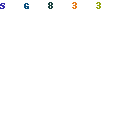Morse Code Practice Oscillator
by Tony van Roon```
Parts List
R1 = 3K3               IC1 = 555 Timer IC
R2 = 15K                S1 = Morse Keyer
P1 = 500 ohm
C1 = 0.047uf (47nF)
C2 = 0.01uF (10nF)
C3 = 4.7uF/16v
```